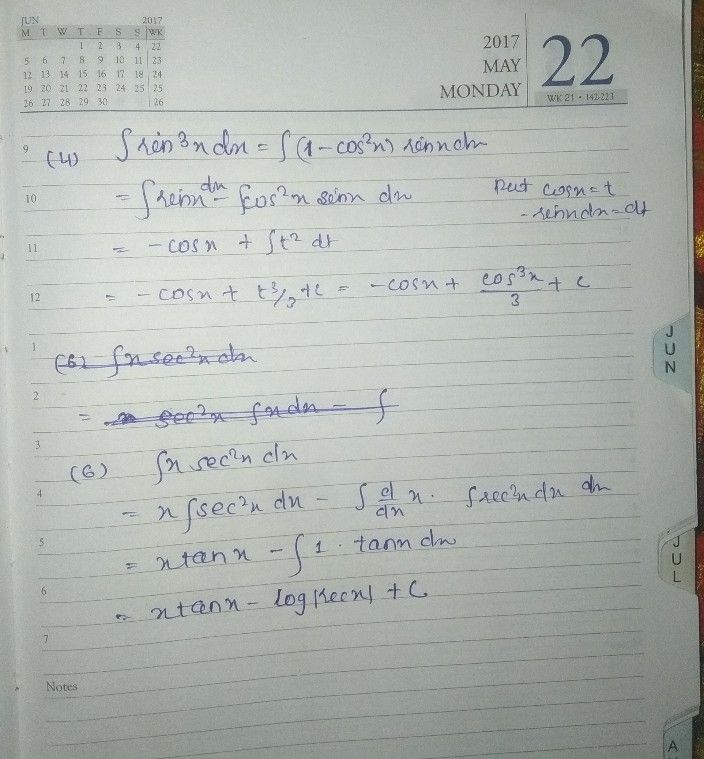Symbol
Problem$-\left(4\right)$ Evaluate: $\int sin^{3}xdx$ $\left(5\right)$ Evaluate $:$ $\int \dfrac {sinx} {1+cos^{2}x}dx$ $\left(6$ Evaluate $:$ $\int$ $xsec^{2}xdx$ $\pi 12$ $\left(7\right)$ Evaluate $:$ $\int \dfrac {cos^{2}x} {1-sinx}dx$